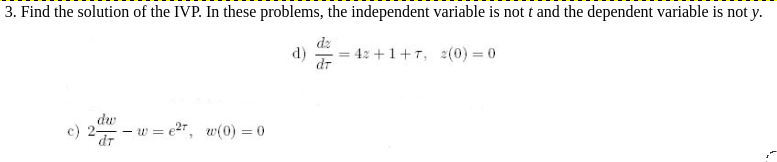Home / Answered Questions / Other / 3-find-the-solution-of-the-ivp-in-these-problems-the-independent-variable-is-not-t-and-the-dependent-aw573

# (Solved): 3. Find The Solution Of The IVP. In These Problems, The Independent Variable Is Not T And The Depend...3. Find the solution of the IVP. In these problems, the independent variable is not t and the dependent variable is not y. = 4: +1+T, 2(0) = 0 c) 204 â€“ w = 27, w(0) = 0

We have an Answer from Expert